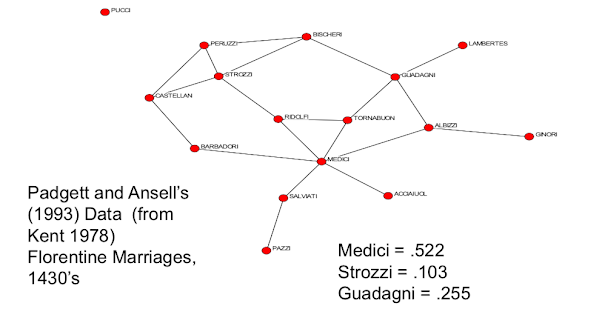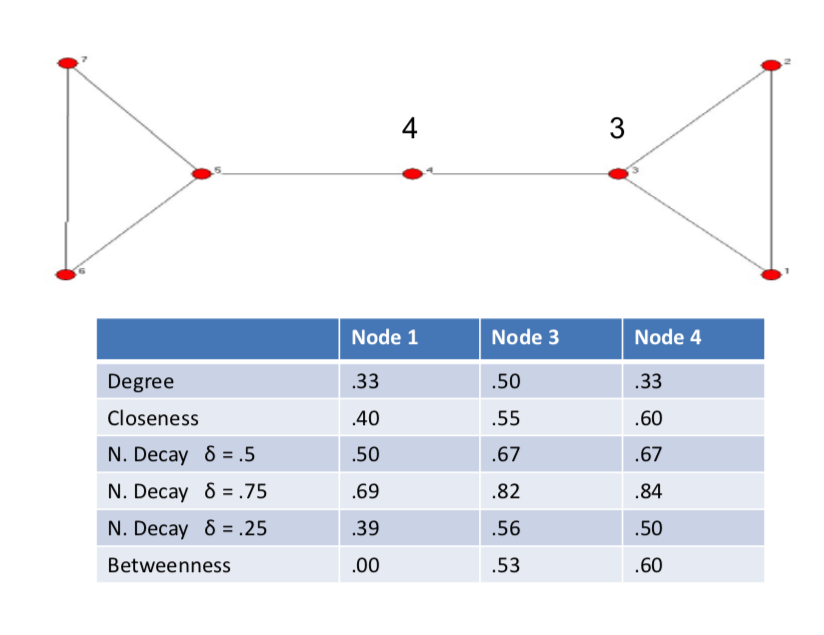# Simplifying the Complexity¶

• Global patterns of networks

• degree distributions, path lengths...
• Segregation Patterns

• node types and homophily
• Local Patterns

• Clustering, Transitivity, Support...
• Positions in networks

• Neighborhoods, Centrality, Influence...

# Position in Network¶

• How to describe individual characteristics?
• Degree
• Clustering
• Distance to other nodes
• Centrality, influence, power...???

# Degree Centrality¶

so the idea of how to describe a position in a network you know, there's different aspects of individual characteristics, some of which is how connected it is. How clustered its friends are. distance to other nodes. but more generally, trying to capture centrality, influence, and power are going to build on specific definitions which, keep track of a node's position. So, in terms of looking at nodes centrality. the most basic measure in just trying to figure out how important a node is, or how influential it is and so forth. It's just how connected it is and that's captured directly by degree. So degree captures some notion of connectedness of a node. And you know, in order to make it a measure between zero and one, we can just keep track of dividing through by n - 1 the most possible links I could have and then what fraction of people am I connected to compared to how many I could be connected to.

• How "connected" is a node?
• degree captures connectedness
• normalize by n-1 - most possible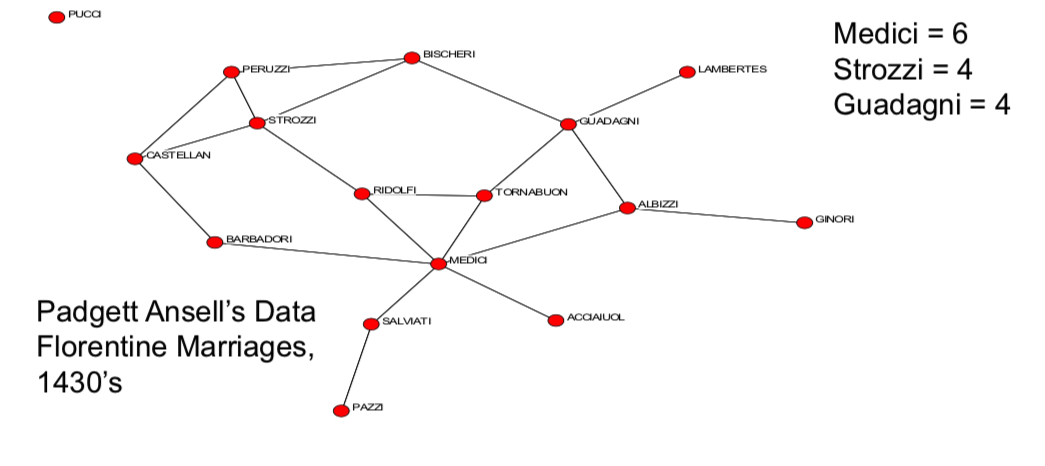# Centrality, Four different things to measure¶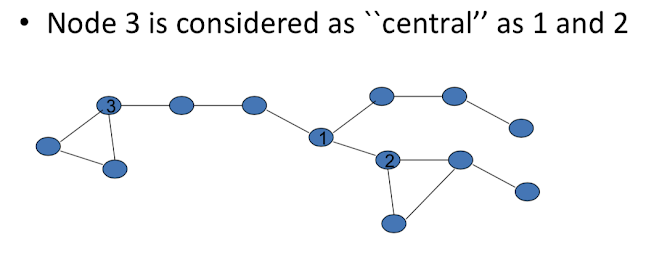Nodes 1, 2 and 3 have the same degree, so how do we measure the position of these nodes in network, or central you are in a deeper sense.

In order to get at things like centrality, we'll have different types of things that we can think about capturing:

• Degree - basic connectedness
• Closeness, Decay - ease of reaching other nodes
• Betweenness - role as an intermediary, connector
• role as an intermediary or connector, so are do other pairs of nodes have to go through me in order to reach themselves? that's a very different concept then thinking how close I am to somebody else. this is saying am I as important as connector of other individuals.
• Influence, Prestige, Eigenvectors -
• "not what you know, but who you know..."
• capture the idea that you are important if your friends are important

# Closeness¶

• Closeness centrality: $$(n-1) / \sum_i I(i, j)$$ where $I(i, j)$ is the length of the shorest path between two nodes i and j

then we can sum across all j, so how far am I away from all the other nodes? n-1 over this, so it keeps track of sort of relative nodes.

• relative distances to other nodes

• scales directly with distance - twice as far from everybody makes me half as central. so is I double all these things, I'm going to get half. if I quadruple them, then I'll get a quarter.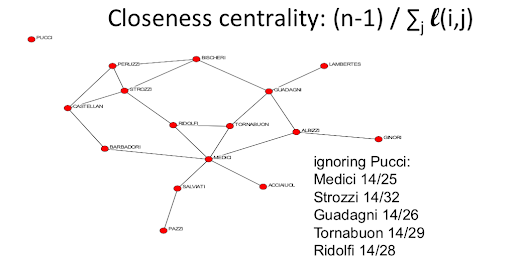# Decay Centrality¶

another measure that we could use instead, is what's known as decay centrality. And this is, designed to capture the idea that, what I might get is value from being connected or indirectly connected to other nodes. So I might have some value from a friend a different value from a friend of a friend, and so forth. And so the idea is that there's going to be some $\delta$ factor which is, generally less than 1 and bigger than 0. And the centrality then of a node i under this decay notion, is going to be :

$$C_i^d(g) = \sum_{j \ne i} \delta^{I(i, j)}$$

another measure that we could use instead, is what's known as decay centrality. And this is, designed to capture the idea that, what I might get is value from being connected or indirectly connected to other nodes. So I might have some value from a friend a different value from a friend of a friend, and so forth. And so the idea is that there's going to be some $\delta$ factor which is, generally less than 1 and bigger than 0. And the centrality then of a node i under this decay notion, is going to be $C_i^d(g) = \sum_{j \ne i} \delta^{I(i, j)}$.

So if I'm a direct friend I get a $\delta$. If I'm an indirect friend, distance 2 from somebody, I get $\delta^2$. distance 3 $\delta^3$. So if this were 0.5 then we're going to get 0.25 here. and, and, and so forth. 0.125. if this were 0.9 then these numbers would be much closer to each other. If this was, you know, 0.05 then this would be 0.0025 and so forth and so it would scale more dramatically. So as $\delta$ becomes near 1, then this just sort of counts all the people that I can reach indirectly.

• $\delta$ near 1 becomes component size

• then this just sort of counts all the people that I can reach indirectly
• $\delta$ near 0 becomes degree

• then this is just goting to become degree centrallity. all it's going to do is really emphasize the direct connections and all the other ones are going to be much smaller.
• $\delta$ in between decaying distance measure

• weigthts distance exponentially

So you can think of varying this $\delta$, as sort of how much do I think of it being important to be close to many people, or of how much do I get from indect connections of different varying lengths.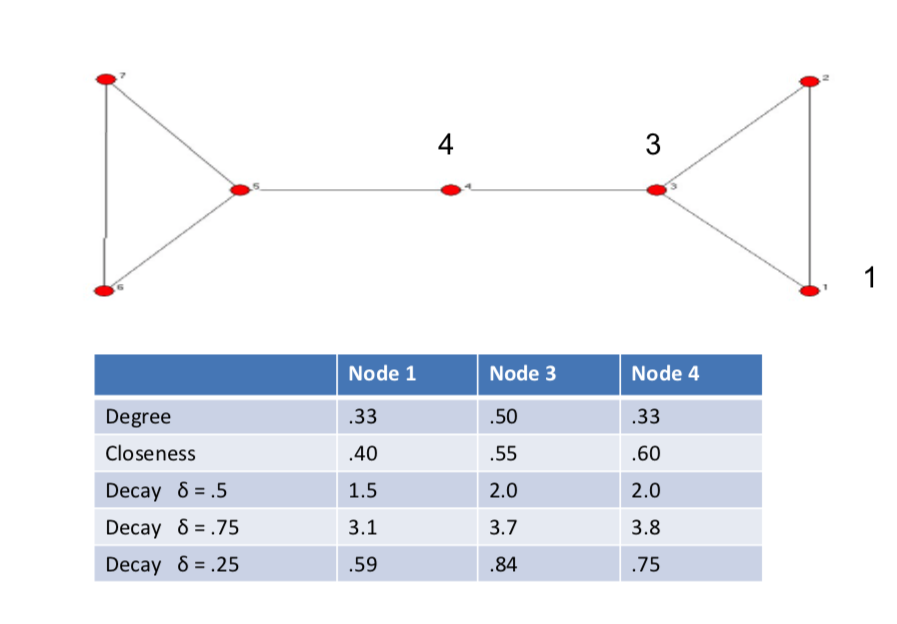# Normalize: Decay Centrality¶

$$C_i^d(g) = \sum_{j \ne i} \delta^{I(i, j)} / ((n - 1)\delta)$$

$(n-1)\delta$ is the lowest decay possible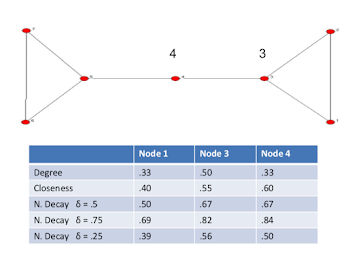# Betweenness (Freeman) Centrality¶

• $P(i, j)$ number of geodesics btwn i and j
• $P_k(i,j)$ number of geodesics btwn i and j that k lies on
• $\sum_{i, j \ne K}[P_k(i,j)/P(i,j)] / [(n-1)(n-2)/2]$